## Objective

Main objective of this tutorial is to explain how to display projectile trajectory path in Unity 3D with code sample (example).

## Step 1 Understand About Projectile

A projectile is an object upon which the only force acting is gravity. There are a variety of examples of projectiles. A cannonball shot from a cannon, a stone thrown into the air, or a ball that rolls off the edge of the table are all projectiles. These projectiles follow curved paths called trajectories. When air resistance is neglected the curved paths are parabolic in shape.

## Step 2 Types Of Projectile Motion

Many projectiles not only follow a vertical motion, but also follow a horizontal motion. That is, as they move upward or downward they are also moving horizontally. There are the two components of the projectile's motion - horizontal and vertical motion.

### 2.1 Vertical Motion

In Vertical Motion, the gravity acts on objects and gives them negative acceleration “-9.8m/s²” (gravity of earth). This means that, velocity of objects decreases "-9.8m/s²" in each second. The velocity of the free falling object is V=g*t.

If we have initial velocity then, equation of velocity of falling object: V = Vi + g*t where acceleration is -9.8m/s² The distance in free fall is calculated by the equation:

• DistanceTraveled = 1/2*g*t*t;

As in the velocity case our distance is calculated considering the initial velocity of the object by the formula;

• DistanceTraveled = Vit - 1/2*g*t*t;

Distance is subtracted because direction of g is downward.

### 2.2 Horizontal Motion

In Horizontal Motion, motion will be constant because there is no force acting on objects in horizontal direction. Thus, the X component of velocity is constant and acceleration in X direction is zero. The equation that is used to calculate distance and velocity is given below.

• DistanceTraveled = v*t;

## Step 3 Implementation

The following simple c# code will display the projectile trajectory path of ball when it will thrown from cannon.

### Note

Add following script on cannon object. Create prefebs for ball and trajectory point which will be instantiate runtime. Ball must have collider and rigidbody.

Screenshots:

•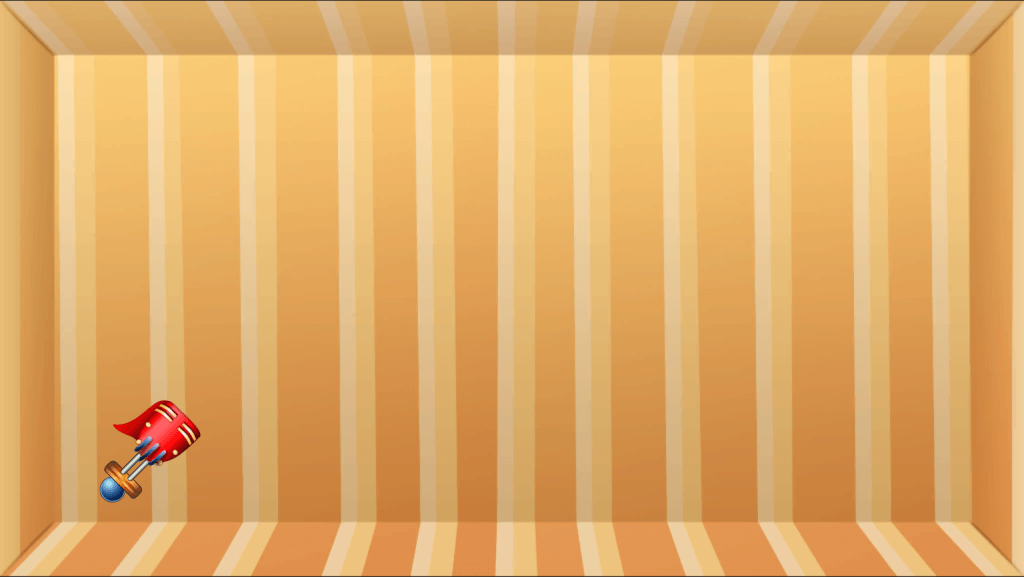•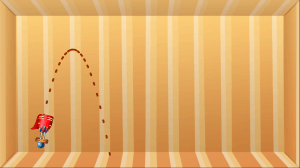•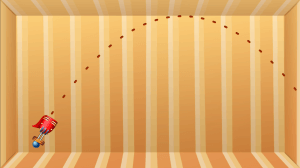•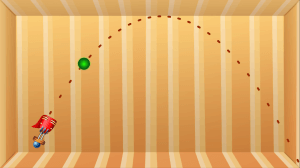•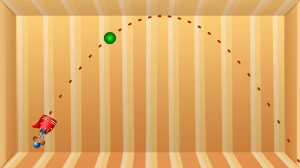•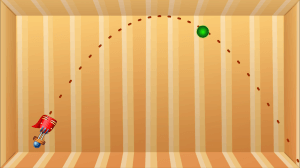•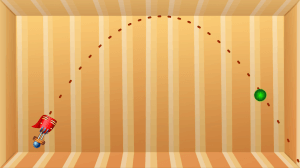### 3.1 Cannon Script

``````using UnityEngine;
using System.Collections;
using System.Collections.Generic;

public class CannonScript : MonoBehaviour
{
// TrajectoryPoint and Ball will be instantiated
public GameObject TrajectoryPointPrefeb;
public GameObject BallPrefb;

private GameObject ball;
private bool isPressed, isBallThrown;
private float power = 25;
private int numOfTrajectoryPoints = 30;
private List trajectoryPoints;
//---------------------------------------
void Start ()
{
trajectoryPoints = new List();
isPressed = isBallThrown =false;
//   TrajectoryPoints are instatiated
for(int i=0;i<numOfTrajectoryPoints;i++)
{
GameObject dot= (GameObject) Instantiate(TrajectoryPointPrefeb);
dot.renderer.enabled = false;
trajectoryPoints.Insert(i,dot);
}
}
//---------------------------------------
void Update ()
{
if(isBallThrown)
return;
if(Input.GetMouseButtonDown(0))
{
isPressed = true;
if(!ball)
createBall();
}
else if(Input.GetMouseButtonUp(0))
{
isPressed = false;
if(!isBallThrown)
{
throwBall();
}
}
// when mouse button is pressed, cannon is rotated as per mouse movement and projectile trajectory path is displayed.
if(isPressed)
{
Vector3 vel = GetForceFrom(ball.transform.position,Camera.main.ScreenToWorldPoint(Input.mousePosition));
transform.eulerAngles = new Vector3(0,0,angle);
setTrajectoryPoints(transform.position, vel/ball.rigidbody.mass);
}
}
//---------------------------------------
// Following method creates new ball
//---------------------------------------
private void createBall()
{
ball = (GameObject) Instantiate(BallPrefb);
Vector3 pos = transform.position;
pos.z=1;
ball.transform.position = pos;
ball.SetActive(false);
}
//---------------------------------------
// Following method gives force to the ball
//---------------------------------------
private void throwBall()
{
ball.SetActive(true);
ball.rigidbody.useGravity = true;
isBallThrown = true;
}
//---------------------------------------
// Following method returns force by calculating distance between given two points
//---------------------------------------
private Vector2 GetForceFrom(Vector3 fromPos, Vector3 toPos)
{
return (new Vector2(toPos.x, toPos.y) - new Vector2(fromPos.x, fromPos.y))*power;
}
//---------------------------------------
// Following method displays projectile trajectory path. It takes two arguments, start position of object(ball) and initial velocity of object(ball).
//---------------------------------------
void setTrajectoryPoints(Vector3 pStartPosition , Vector3 pVelocity )
{
float velocity = Mathf.Sqrt((pVelocity.x * pVelocity.x) + (pVelocity.y * pVelocity.y));
float angle = Mathf.Rad2Deg*(Mathf.Atan2(pVelocity.y , pVelocity.x));
float fTime = 0;

fTime += 0.1f;
for (int i = 0 ; i < numOfTrajectoryPoints ; i++)
{
float dx = velocity * fTime * Mathf.Cos(angle * Mathf.Deg2Rad);
float dy = velocity * fTime * Mathf.Sin(angle * Mathf.Deg2Rad) - (Physics2D.gravity.magnitude * fTime * fTime / 2.0f);
Vector3 pos = new Vector3(pStartPosition.x + dx , pStartPosition.y + dy ,2);
trajectoryPoints[i].transform.position = pos;
trajectoryPoints[i].renderer.enabled = true;
trajectoryPoints[i].transform.eulerAngles = new Vector3(0,0,Mathf.Atan2(pVelocity.y - (Physics.gravity.magnitude)*fTime,pVelocity.x)*Mathf.Rad2Deg);
fTime += 0.1f;
}
}
}``````

Every mobile development project has to face challenges, Only the team which has experience and knows how to overcome them can get success. Contact us if you wish develop iOS, Android or Windows games in Unity. We are one of the best Game Development Company in India.I am professional Game Developer, developing games in cocos2d(for iOS) and unity(for all platforms). Games are my passion and I aim to create addictive, high quality games. I have been working in this field since 1 year.